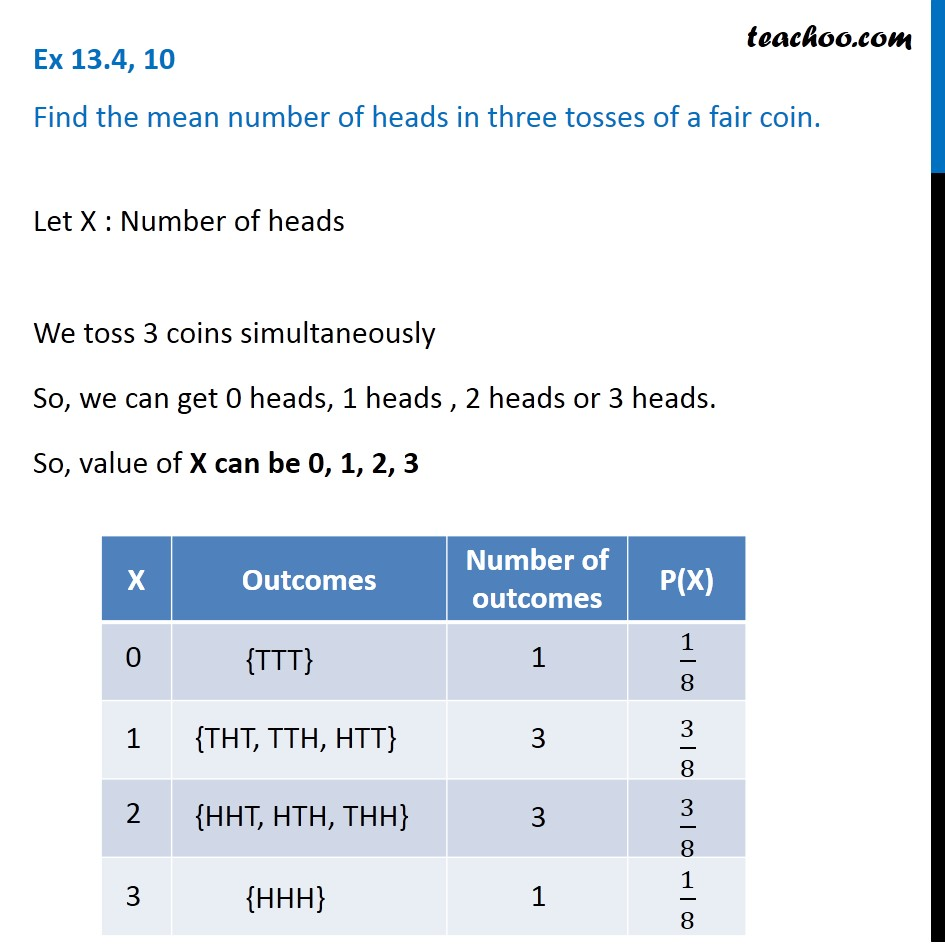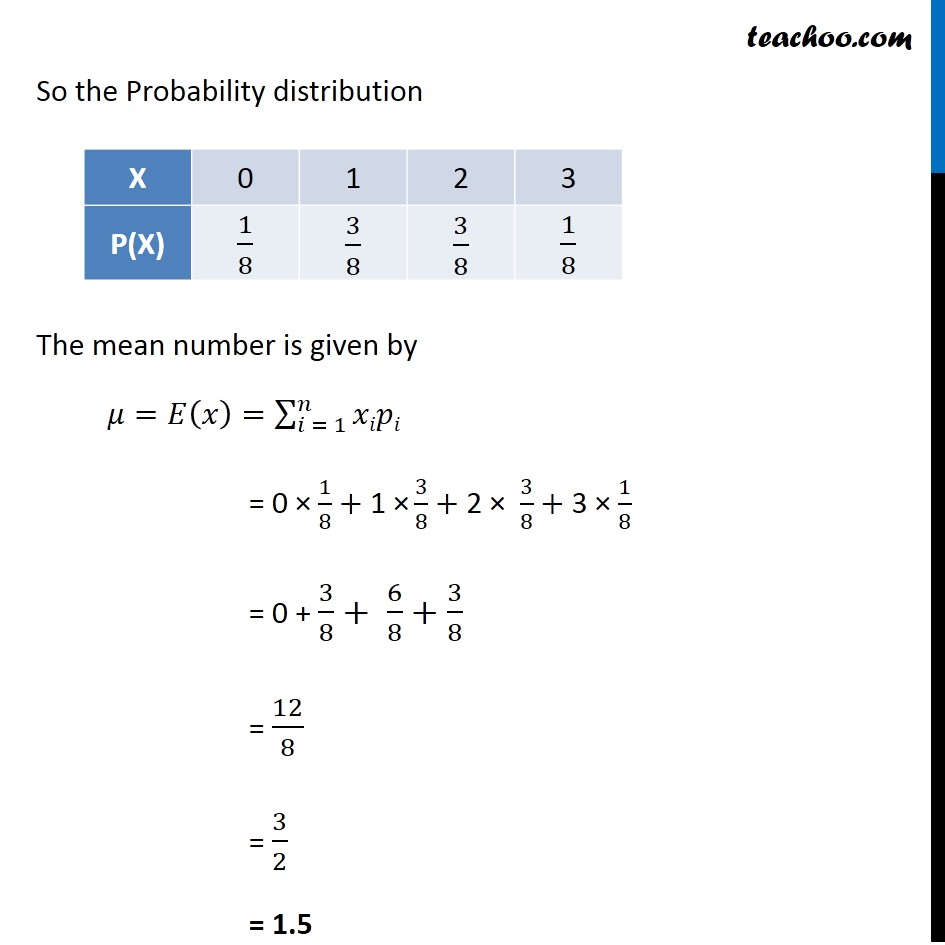Probability Distribution

Chapter 13 Class 12 Probability
Serial order wiseLearn in your speed, with individual attention - Teachoo Maths 1-on-1 Class

### Transcript

Question 10 Find the mean number of heads in three tosses of a fair coin.Question 10 Find the mean number of heads in three tosses of a fair coin.So the Probability distribution The mean number is given by 𝜇=𝐸(𝑥)=∑2_(𝑖 = 1)^𝑛▒𝑥𝑖𝑝𝑖 = 0 × 1/8+"1 ×" 3/8+ 2 × 3/8+ 3 × 1/8 = 0 + 3/8+ 6/8+3/8 = 12/8 = 3/2 = 1.5